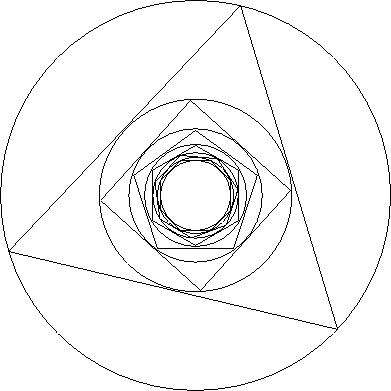# Inscribed To Infinity

Geometry Level 4Take a circle of radius 1. Inscribe a regular triangle in this circle. Inscribe a circle in this triangle. Inscribe a square in it. Inscribe a circle, regular pentagon, circle, regular hexagon and so forth.

What is the radius of the circle formed when the process is carried forever (the radius of the limiting circle)?

Give the answer correct to 3 decimal places.

×